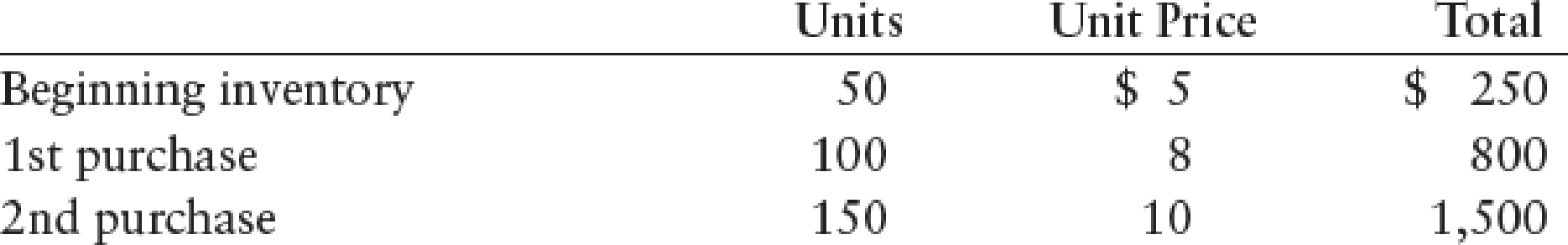Chapter 13, Problem 3CE

Chapter
Section
Textbook Problem

Use the following information to compute cost of goods sold under the FIFO and LIFO inventory methods. The firm sold 200 units.To determine

Compute the cost of goods sold under the FIFO and LIFO inventory methods.

Explanation

First-in-First-Out (FIFO): In First-in-First-Out method, the first purchased items are sold first. The value of the ending inventory consists of the recently purchased items.

Calculate the cost of goods sold under FIFO method:

Step 1: Calculate the total cost and units of goods available for sales.

 Calculation of Goods Available for Sales Details Number of Units Rate per unit ($) Total Cost ($) Beginning balance 50 5 250 Add: Purchases 1st Purchases 100 8 800 2nd Purchases 150 10 1,500 Total Goods available for Sale 300 $2,550 Less: Sales: 200 Ending Inventory 100 Table (1) Step 2: Calculate the cost of ending inventory.  Calculation of Cost of Ending Inventory Details Number of Units Rate per Unit ($) Total Cost ($) 2nd Purchases 100 10 1,000 Ending Inventory 100$1,000

Table (2)

Step 3: Calculate the cost of goods sold under FIFO method...

Still sussing out bartleby?

Check out a sample textbook solution.

See a sample solution

The Solution to Your Study Problems

Bartleby provides explanations to thousands of textbook problems written by our experts, many with advanced degrees!

Get Started

Find more solutions based on key concepts